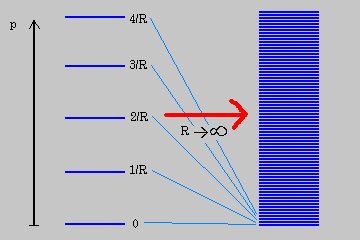## Wednesday, January 04, 2006

### KK Tower

Like many people who devote their time to understanding the nature of the cosmo and the micro perspective of the world around us, these things have their own motivational packages which move to further rquired comprehensions. In that, one needs to further educateas to what they are talking about.

It's definitiely not easy, but I am trying, and devote a lot of time to this regardless of what schooling is required, it is not my intent to send people down the wrong paths, or, no paths at all, before I have investigated the terrain as best I can.

Mountains can give persepctive where sitting in the valleys circumspect what the greater can be?

KK Tower

What is it?Kaluza-Klein theory(Wiki 4 Jan 2006)

A splitting of five-dimensional spacetime into the Einstein equations and Maxwell equations in four dimensions was first discovered by Gunnar Nordström in 1914, in the context of his theory of gravity, but subsequently forgotten. In 1926, Oskar Klein proposed that the fourth spatial dimension is curled up in a circle of very small radius, so that a particle moving a short distance along that axis would return to where it began. The distance a particle can travel before reaching its initial position is said to be the size of the dimension. This extra dimension is a compact set, and the phenomenon of having a space-time with compact dimensions is referred to as compactification.

Kaluza-Klein theory is a model which unifies classical gravity and electromagnetism. It was discovered by the mathematician Theodor Kaluza that if general relativity is extended to a five-dimensional spacetime, the equations can be separated out into ordinary four-dimensional gravitation plus an extra set, which is equivalent to Maxwell's equations for the electromagnetic field, plus an extra scalar field known as the "dilaton". Oskar Klein proposed that the fourth spatial dimension is curled up with a very small radius, i.e. that a particle moving a short distance along that axis would return to where it began. The distance a particle can travel before reaching its initial position is said to be the size of the dimension. This, in fact, also gives rise to quantization of charge, as waves directed along a finite axis can only occupy discrete frequencies.

Kaluza-Klein theory can be extended to cover the other fundamental forces - namely, the weak and strong nuclear forces - but a straightforward approach, if done using an odd dimensional manifold runs into difficulties involving chirality. The problem is that all neutrinos appear to be left-handed, meaning that they are spinning in the direction of the fingers of the left hand when they are moving in the direction of the thumb. All anti-neutrinos appear to be right-handed. Somehow particle reactions are asymmetric when it comes to spin and it is not straightforward to build this into a Kaluza-Klein theory since the extra dimensions of physical space are symmetric with respect to left-hand spinning and r-hand spinning particles.

So in order to get to the summation, views of hidden dimenisons had to be mathematically described for us, so a generalization here would suffice in the following diagram.Now, not having the room to explain, and having linked previous information on extension of KK theory, I wondered about the following. If we understood well, the leading perspective that lead us through to the dynamical realizations, then the road Gauss and Reimann lead us to would help us to understand the visualization materializing by the calorimeter disciptions of each energy placement harmonically describing each particle's value? Even in a empty space, there seems to be something of a harmonical consideration?

If one understood well enough about the direction of discernation of early universe consideration and microstates, then such questions would have been of value in the ideas of topological considerations?